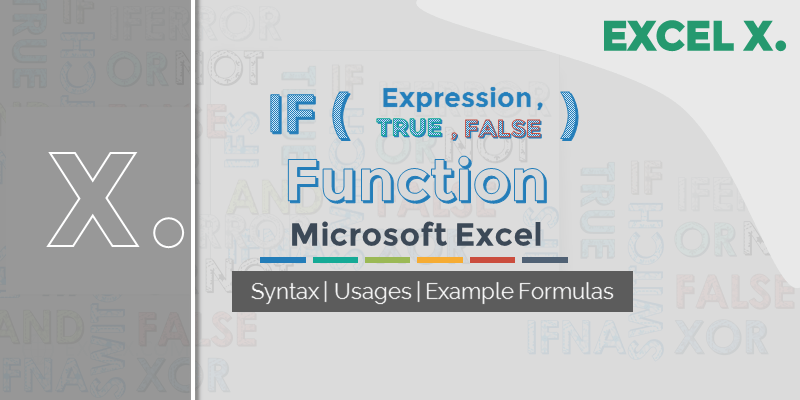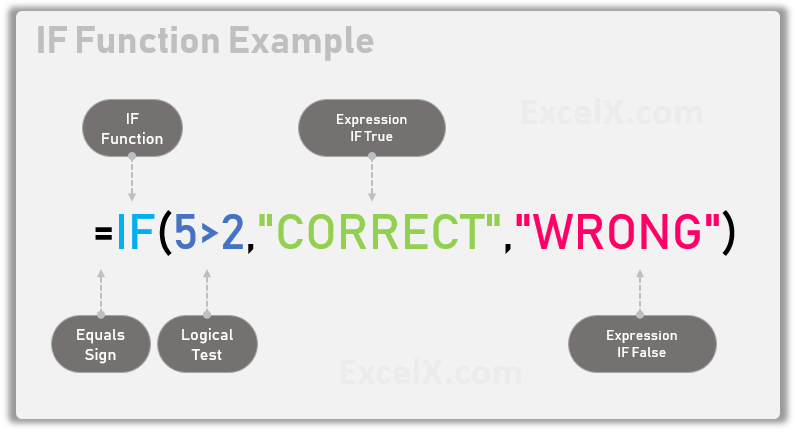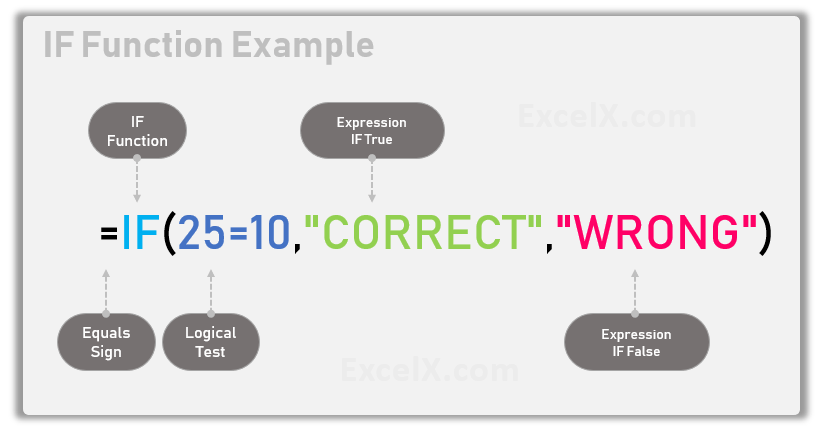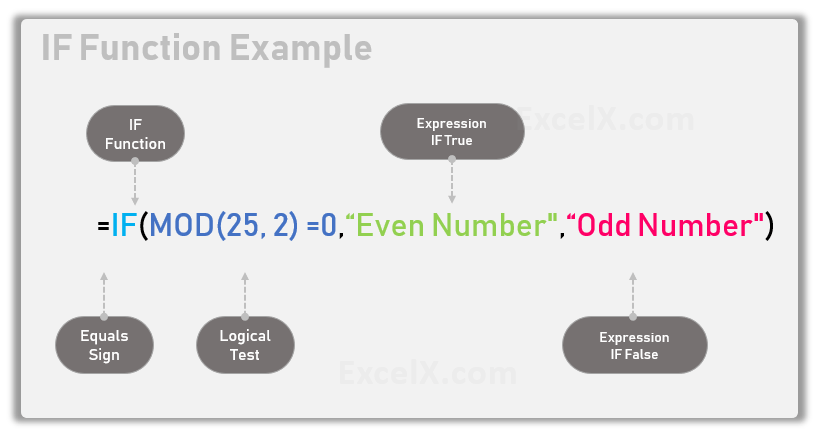IF Function helps to evaluate the logical expression and return the values for both Boolean results. We can specify two values or expressions to return when the logical expression is TRUE or FALSE. Let us see the syntax of IF Function and real-time examples on IF Function for better understanding.

## Syntax of IF Function

If Function Evaluate the first argument and resolves the second argument if the first argument returns TRUE, it resolves the third argument if the first argument returns FALSE.

=IF(logical_test,[value_if_true],[value_if_false])=IF(Expression to Evaluate,Expression If True,Expression If False)

## Simple Examples of Excel IF Function

Here the simple examples for easily understanding IF Function. Copy these example to any celll in the Excel Sheet and see the result for cleary understanding the IF Function.

Example 1: The following example returns “CORRECT” based the given expression.

=IF(5>2,”CORRECT”,”WRONG”)

returns “CORRECT”

IF Function Checks the first argument (5>2) and it returns TRUE. It will evaluate the second argument (“CORRECT”) as it the condition is True.1. =IF(): Statement to Call Excel IF Function
2. 5>2: is the logical test if 5 is greater than 2
3. “CORRECT”: value if the logical test returns TRUE
4. “WRONG”: value if the logical test returns FALSE

Copy the above example and paste in any Cell, this example IF Function returns “CORRECT”.

Example 2: The following example returns “WRONG” based the given expression.

=IF(25=10,”CORRECT”,”WRONG”)

returns “WRONG”

IF Function Checks the first argument (25=10) and it returns FALSE. It will evaluate the third argument (“WRONG”) as it the condition is False.1. =IF(): Statement to Call Excel IF Function
2. 25=10: is the logical test to check if 25 is equals to 10
3. “CORRECT”: value if the logical test returns TRUE
4. “WRONG”: value if the logical test returns FALSE

Copy the above example and paste in any Cell, this example IF Function returns “WRONG”.

Example 3: The following example checks if the given value is even or odd number.

=IF(MOD(25, 2) =0,”Even Number”,”Odd Number”)

returns “Odd Number”

IF Function Checks the first argument [MOD(25, 2) =0] and it returns FALSE. It will evaluate the third argument (“Odd Number”) as it the condition is False.1. =IF(): Statement to Call Excel IF Function
2. MOD(25, 2) =0: is the logical test to check if 25 MOD 2 is equals to 0
3. “Even Number”: value if the logical test returns TRUE
4. “Odd Number”: value if the logical test returns FALSE

Copy the above example and paste in any Cell, this example IF Function returns “Odd Number”.

## Excel IF Function – Practical Examples:

Here are the practical examples of IF Function. IF function is on of the most commonly used Excel Function to check the logical test and nested IF Functions. We can combine the IF Function with Other Excel Function to build the complex expressions and formulas.

##### IF Function to Compare This Year and Last Year Values:

The following Example shows how to compare the sales values of Current Year and Last Year. We have provide Deparment wise data in the sheet with This Year Data in Column B and This Year Data in Collumn C. And logical test is performed in Column D to check if the Column C values are greater the Column B Values.=IF(C2>B2,”PROFIT”,”LOSS”)

IF Function to compare the values in two columns.

You can copy this formula and paste in the Cell D2 and double click to fill the data range.

1. =IF(): Statement to Call Excel IF Function
2. C2>B2: is the logical test to check if 25 MOD 2 is equals to 0
3. “Profit”: value if the logical test returns TRUE
4. “Loss”: value if the logical test returns FALSE

#### If Function to Compare Sum of the Column Values

The following IF Formula compare the Sum of Two Columns in Excel Sheet. This will check if Sum of Column C (Range C2:C12) values are greater than Sumn of Column B (Range B2:B12).=IF(SUM(C2:C11)>SUM(B2:B11),”Profit”,”Loss”)

IF function to compare sum of two ranges in Excel.

1. =IF(): Statement to Call Excel IF Function
2. SUM(C2:C11)>SUM(B2:B11): is the logical test to check if 25 MOD 2 is equals to 0
3. “Profit”: value if the logical test returns TRUE
4. “Loss”: value if the logical test returns FALSE

If in Excel have verity of forms and applications. We can use IF formula to check the Boolean condition. We can return some value if the condition is true and some other value if not true or false. Let us see the in-depth details about If in Excel.

## If Formula in Excel

We can use the If formula in Excel to check if a condition is meeting certain scenario. For example, we can check if the given value is equals to an integer. We can use If formula to compare two strings. We can return the values based on the Boolean result of If formula in Excel. The following example check if the given value is greater than 10 or not. And return the message based on the result.

`=If(25>10,"Given Value is bigger than 10","Given Values is Not Bigger Than 10")`

## If Formula to check Cell Values

We can check the cell value using If formula and perform the calculations based on the result. Here is a simple example in Cell B1 to check if a cell (A1) is less than or equals to 1000 or not. And add 500 if the values is less than 1000.`=IF(A1<1000,A1+500,A1)`

If formula checks the condition (A1<1000) and add 500 if the cell value is less than 1000,  it will return the same value if the condition fails.

## If Formula to check all values in a Column

We can check the values in a column and perform certain calculation based on the result. Let us see an example to check if any value in column B is equals to zero and flag the respective cell in Column C.`=IF(B1=0,"Zero","")`

You can put this formula in C1 and fill-down upto rows of B1.

1. Greg October 29, 2019 at 9:21 am - Reply

Thank you for this easy to understand tutorial on Excel If function. I can say that this is the best way and simple approach to learn IF formula with strong understanding of fundamentals of Excel IF function.

2. Anna October 29, 2019 at 9:27 am - Reply

Yes, agree with you!
I am completely impressed with the way of explaining the If function with suitable and real-time examples.

Excellent! Good Job!! Keep it Up!

3. Jose Valle June 22, 2022 at 8:58 pm - Reply

how can I make this formula work =MOD(E2-B2-D2+C2,1)*24,IF(G2=”Yes”,F2+0.5,IF(G2=”No”,\$F\$2)) what I’m I missing or need to do

• PNRao July 1, 2022 at 2:50 am - Reply

Comma(,) following number 24. Please describe your question in detailed. Just to inform you, the Mod Value with divisor 1 always return 0.